If the set W is a vector space, find a set S of vectors that spans it. Otherwise, state that W is not a vector space. W is the set of all ve

Question

If the set W is a vector space, find a set S of vectors that spans it. Otherwise, state that W is not a vector space. W is the set of all vectors of the form [a – 4b 5 4a + b -a – b], where a and bare arbitrary real numbers.
a. [1 5 4 -1], [-4 0 1 -1]
b. [1 0 4 -1], [-4 5 1 -1]
c. [1 0 4 -1], [-4 0 1 -1], [0 5 0 0]
d. Not a vector space

in progress 0
5 months 2021-08-16T05:03:34+00:00 1 Answers 25 views 0

Choice d. The set of vectors: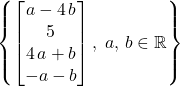isn’t a vector space over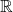.

Explanation:

Let a set of vectors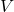to be a vector field over some field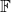(for this question, that “field” is the set of all real number.) The following must be true:

• The set of vectorsincludes the identity element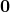. In other words, there exists a vector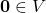such that for all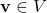,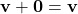.
•should be closed under vector addition. In other words, for all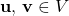,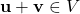.
•should also be closed under scalar multiplication. In other words, for alland all “scalar”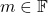(in this question, the “field” is the set of all real numbers, so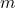can be any real number,)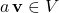.

Note that in the general form of a vector in, the second component is a always non-zero. Because of that non-zero component,

Assume by contradiction thatis indeed a vector field. Therefore, it should contain a zero vector. Letdenote that zero vector. For all,.

Using the definition of set:, there exist real numbers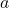and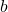, such that: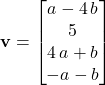.

Hence,is equivalent to: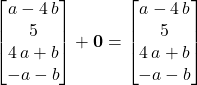.

Apply the third property thatis closed under scalar multiplication.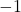is indeed a real number. Therefore, if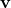is in

Therefore: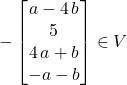.

Apply the second property and add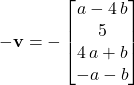to both sides of. The left-hand side becomes: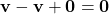.

The right-hand side becomes: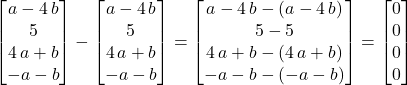.

Therefore: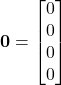.

However,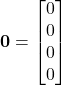isn’t a member of the set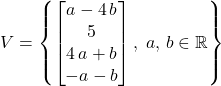. That’s a contradiction, becausewas supposed to be part of.

Hence,isn’t a vector space by contradiction.